﻿ A Review of Software Packages for Structural Equation Modeling: A Comparative StudyPublications are Open
Access in this journal
Article Versions
Export Article
• Normal Style
• MLA Style
• APA Style
• Chicago Style
Original Article
Open Access Peer-reviewed

### A Review of Software Packages for Structural Equation Modeling: A Comparative Study

Ahmed A. El-Sheikh, Mohamed R. Abonazel , Noha Gamil
Applied Mathematics and Physics. 2017, 5(3), 85-94. DOI: 10.12691/amp-5-3-2
Published online: August 04, 2017

### Abstract

Structural equation modeling (SEM) is a widely used statistical method in most of social science fields. Similar to other statistical methods, the choice of the appropriate estimation methods affects the results of the analysis, thus it was of importance to review some of SEM software packages and the availability of different estimation methods in these packages. Therefore, in this paper five SEM software packages (AMOS, LISREL, and three packages in R) dealing with SEM analysis were reviewed to guide the researcher about the usage of each package. Moreover, an empirical study was presented to assess the performance of different estimation methods under the existence of missing data. The results showed that full information maximum likelihood (FIML) was the best estimation method to deal with different missingness rates.

### 1. Introduction

Structural Equation modeling (SEM) applications are widely spread nowadays due to the need of this methodology in most of the social science studies. As a result, SEM statistical packages are rapidly growing to serve in this matter. The mostly used packages for SEM studies are Mplus, EQS, Amos, LISREL, and several packages in R. It was found that assessing the performance of structural equation modeling estimation methods through a mathematical derivation is not always enough, as the estimation part is imposed to some limitations/restrictions that occur only in reality and cannot be predicted through derivation. It was essential here to examine the performance of these methods through a real data application as well, thus, it was planned to conduct an application of SEM.

In this paper, five SEM software packages (AMOS, LISREL, and three packages in R) are reviewed to provide the researcher with the basic guidelines about each package. Moreover, an application of SEM on a standard dataset is introduced, where the performance of different estimation methods explained in statistical literature will be examined under the characteristics of the used dataset and used model.

The paper is organized as follows. Section 2 presents the characteristics of the used model. Section 3 presents a brief comparative review between the five SEM software packages. Section 4 discusses the advantages of R-packages along with an explanation on how the lavaan package is used. In Section 5, we present an empirical study for assessing the performance of different estimation methods under the existence of missing data, where different rates of missingness on the priory used standard dataset are imposed. Finally, Section 6 offers the concluding remarks.

### 2. The SEM Model

In general, the SEM model expresses the relationship between indicators and latent variables; it can be expressed as follows: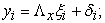(1)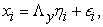(2)

where, model (1) is the measurement model of the exogenous latent variables with manifest variable vector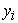on the latent exogenous variables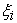,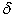is the measurement error in exogenous indicators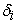. While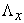is a matrix of factor loadings relating indicators to the latent exogenous variable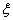, with the errors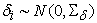. Finally,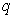represents the number of indicators of latent exogenous variables. Thus, model (1) can be written in a matrix form as: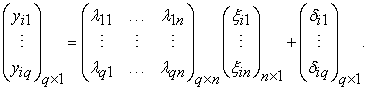Model (2) includes indicator variables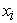in subject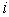considered manifestation of latent endogenous variables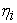, and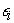is the measurement errors in endogenous variables, where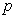represents the number of indicators of latent endogenous variables. While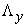is a matrix of factor loadings relating indicators to the latent variable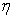, with the errors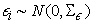. Thus, model (2) can be written in a matrix form as: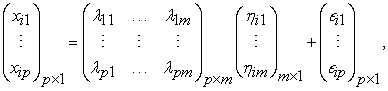where,andare independent normally distributed.

### 3. Software Packages of SEM

The structure of this section will be adopted briefly by the structure of Byrne 6; it will only present a comparative review between AMOS, LISREL, and R.

3.1. Model Specification

AMOS

AMOS has a very interesting feature developed within the Microsoft Windows interface; it allows researchers to either specify the model with drawing a path diagram representing the relationships between variables through AMOS graphics, or to directly write the equation statements through AMOS basics. However, researchers will always opt to use AMOS graphics due to its easiness in identifying the relationships between the variables by using all the tools provided by AMOS graphics that will ever be needed in creating and working with SEM path diagrams 6.

LISREL

The command of LISREL is expressed in an algebraic matrices language which is dominated by the use of Greek letters. However one of the limitations that LISREL program has is that a full latent variable model, based on the analysis of covariance structures, may be defined by a maximum of eight matrices and four vectors; analysis of means structures involves an additional four matrices 5. Thereby, Jöreskog and Sörbom 20 introduced a new command language called SIMPLIS that is intended to create input files and report results in a more user-friendly way. Finally, the SEM model specification in LISREL can be determined through the graphical user interface (GUI) component. It allows the researcher to create a graphical representation and to interactively generate the syntax file by means of a path diagram.

R

R is free open source software that enables the S statistical programming language and computing environment for interactive data analysis 12. Programming language called ‘S’ includes conditionals, loops, user defined recursive functions and input and output facilities. It is growing rapidly and has been extended to a large collection of packages. However, each package is mainly created for analyzing data under specified case, like for example packages that are designed specifically to fit the SEM models. These packages have the capability to fit structural equation in observed and latent variables. The packages that fit the SEM models, each with its own characteristics are sem, OpenMx, and lavaan.

3.2. Model Estimation

The default estimation method in the three software programs is maximum likelihood. However, each program has other available estimation methods that can be shown in Table 1. It is important to note that when we are comparing R we are mainly talking about lavaan, sem, and OpenMx packages available in R.

R-packages include a good mixture of estimation methods as seen in Table 1, thereby, since R is free software and it includes the largest number of available estimation methods; the analysis of the dataset will be done, in this paper, using one of SEM packages available in R software.

3.3. Model Assessment and Goodness-of-fit Indexes

Of a primary importance is to test whether the proposed model fits the data or not. This is mainly examined by the goodness-of-fit indexe discussed in statistical literature such as Olsson et al 26, Kline 21, Hoyle 17, and Byrne 7. The software programs reviewed here provide the same goodness-of-fit indexes, however, they only differ in the way they report them. Thereby, some of these indexes will be discussed and used in our application.

Root Mean Square Error Approximation (RMSEA)

RMSEA is employed as an indicator of empirical/absolute fit 8 with its value ranging from 0 to 1. MacCallum et al 23 suggested an acceptable value of RMSEA to be between 0.05 and 0.08 in order to have a reasonable well fitted model. Its statistic can be expressed as: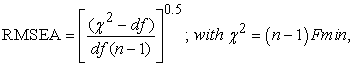where,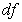is the model degrees of freedom, and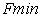is the minimum value of fit function of the estimation method being used.

Despite the popularity of this fit index in SEM studies, it is concluded through simulation studies in the literature that RMSEA does not behave well as it over-rejects the true model under small sample sizes (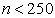), as well as its value might get worse as the number of variables increase in the model. Thereby, SRMR was recommended over RMSEA 19.

Standardized Root Mean Square Residual (SRMR)

SRMR has similar properties of RMSEA index, however it is computed differently, and it also indicates bad fit to the model with higher values of it, while a good fit to the model would be an SRMR value that is close to zero. However, Hu and Bentler 18 suggested a value of SRMR that is less than 0.08 indicates a good fit to the model. Its statistic can be expressed as: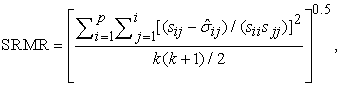where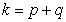,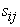are the sample covariances between observed variables, and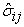are the estimated components of variance-covariance matrix of the error vector of the model.

Comparative Fit Index (CFI)

CFI value ranges between 0 and 1, with value closer to 1 indicating better fit. Hooper et al 15 stated that recent studies suggested a value of CFI above 0.95 in considered an indicator of good model fit or at least 0.90 or above to ensure that the model is correctly specified. The formula used to compute CFI is expressed as: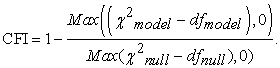Here Max indicates the maximum value of the values given in brackets. The comparison between model’s chi-square and its degrees of freedom is considered as an adjustment for model parsimony. However, if the model is well fitted (having small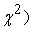there might be a penalty if the model was fitted and it was of a complex model (having several paths leading to using many), however, if thewere similar the entire formula of CFI would be equal to 1.

Tucker-Lewis Index (TLI)

TLI index is introduced by Bentler and Bonett 5 as well, it is also known as Non-Normed Fit index (NNFI). Its value also ranges between 0 and 1 with value closer to 1 indicating better fit to the model, Hu and Bentler 18 suggested a value of 0.95 or higher as an indicator of well fitted model. The formula in which this index is computed can be expressed as: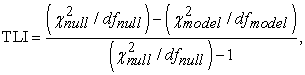where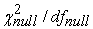is the ratio of chi-square to its degrees of freedom.

3.4. Current Capabilities of SEM Software Packages
3.4.1. Missing Data Treatment

One of the critical issues in SEM that needs to be deeply recognized is the existence of missing values in the dataset being used, as it may yield to misleading results. That is why most of SEM software packages nowadays are addressing this issue by imposing a treatment to the missing cases regardless of the reason for their missingness.

AMOS

AMOS has limited capability in dealing with missing data. It has one single method in treating missingness in AMOS which is FIML estimation method, discussed in details in Allison 3, Gamil 13, and El-Sheikh et al 10.

LISREL

PRELIS program in LISREL is the approach dealing with missing data problem. It provides both listwise and pairwise deletion mechanism of dealing with missingness as well as imputation mechanism. The main limitation of this approach is that if it failed to determine matching case then no imputation is processed, consequently, it will leave the researcher with a proportion of the data that is still missing. A second limitation of this approach is that overlapping between set of variables and the variables researches wish to impute their missing values might occur 6. Thereby, such limitations associated with LISREL negatively influence the privilege of treating missingness.

R

We are mainly referring here to lavaan package. The default act of lavaan in case of missing values in the dataset is to listwise deletion (the estimates are unbiased, although data is lost). If data is missing completely at random (MCAR) or missing at random (MAR), the appropriate estimation method is FIML 28. However, according to Enders and Bandalos 11 study, it was revealed that FIML yielded more efficient results under MCAR than MAR. Fortunately, lavaan package enables the FIML estimation method by specifying the argument missing="ml" or missing="fiml" when calling the fitting action.

3.4.2. Non-normality Treatment

AMOS

AMOS handles the violation of normality assumption via the use of the bootstrap approach. In which this procedure deals with the original sample as if it is the population and do re-sampling from it, where multiple subsample are drawn randomly from the original sample with replacement to this population. This process provides the researcher with efficient investigation about the variability in the parameter estimates and goodness-of-fit indexes, and thereby the values of the parameter estimates are better assessed with more accuracy 6.

LISREL

LISREL mainly deals with the presence of non-normality through using ADF/WLS method that is distribution free in which normality assumption is not in its requirements. First, using PRELIS approach, the data should be adjusted into asymptotic matrix form; the analysis then will be based on this matrix using WLS method 6. However, one important limitation of this method is its sensitivity to sample size, since it was found through simulation studies that WLS method requires large sample size to perform efficiently, otherwise this limitation would hinder its usefulness in handling non-normality.

R

In the lavaan package, three different approaches were implemented to deal with non-normality assumption which are; ADF estimation, scaled test statistics (ML estimation with robust standard errors and a robust test statistic), and bootstrapping (FIML). Similar to LISREL it was mentioned before that ADF implies no normality assumption. And thereby, variables that are skewed do not give misleading results about the standard errors or test statistic if the ADF estimation method was used.

3.4.3. Categorical Data Treatment

AMOS

Until recently AMOS does not have a determined method to deal with categorical data. However, AMOS might consider ordinal categorical data only by assigning numbers to the categorical responses, and then run the analysis by one the chosen estimation methods 4.

LISREL

Categorical data analysis in LISREL is mainly depending on distribution free estimation procedure; however, this method has some restrictive requirements that represent major weakness to dealing with categorical data 6.

R

With categorical exogenous variables, it needs to create dummy variables to run the model as usual, while categorical endogenous variable require special treatment. In SEM framework, two approaches were adapted to deal with categorical data:

i. Limited information approach: Only univariate or bivariate information is used, while the estimation process might be done through two stages, where ML is used in the first stage, and then WLS is used in the last stage. This is best known in Mplus software as mean-adjusted WLS (WLSM) and mean- and variance-adjusted WLS (WLSMV) estimators as discussed in Hox et al 16. So for lavaan to consider categorical variables, it should define them as ordered using the function ordered in the data, frame before running the analysis, and then by default, lavaan will use robust WLS (DWLS with robust standard errors and a scaled-shifted test statistic; which is equivalent to WLSMV estimator in Mplus)

ii. Full information approach: All information is used, and the most practical method in marginal maximum likelihood estimation 28.

### 4. R-packages for SEM

A deeper attention was essential to be given to R software since it will be the used program to analyze and simulate data throughout this dissertation. We will mainly use lavaan package due to its advanced features that are needed in our analysis.

From Table 2, it is shown that lavaan package includes most of the estimation methods, except for the 2SLS that is only included in sempackage. Thereby, if one wishes to estimate any SEM model using the 2SLS method, choosing sem package is a must.

4.1. Lavaan Package

This package provides researchers a free fully open source, but commercially quality package for latent variable modeling. To explore, estimate, and understand a wide variety of latent variable models lavaan has a collection of tools that enable the user to do this. This includes factor analysis, structural equation modeling, longitudinal, multilevel, latent class, item response, and missing data models. It is working on attracting statistician working in the field of SEM to implement new methodologies and achieve new developments, through having a direct access to SEM code 28, 29.

4.2. Advantages of Using Lavaan Package

1. The results that lavaan gives are almost similar to those obtained from other commercial software programs, like Mplus and EQS.A mimic option is included in all the fitting functions of lavaan, to ensure that the results produced by lavaan are comparable to the output of other commercial software programs. (i.e., if mimic="Mplus", lavaan makes an effort to produce output similar to Mplus output. if mimic="EQS", lavaan also makes an effort to produce similar output to that of EQS at least numerically not visually). Thus, the mimic option makes a smooth transition possible from lavaan to one major commercial software program, and back 28.

2. Two problems that researchers always face have received careful attention in lavaan, which are: a) Support for non-normal data. b) Handling of missing data.

3. In lavaan package, models are specified by means of a powerful, easy-to-text-based syntax describing the model, referred to as the lavaan model syntax”. Rosseel 28 gives a description of the most used operators in lavaan model syntax as shown in Table 3, top panel of the table contains the four formula types that can be used to specify a model in the lavaan model syntax. The lower panel contains additional operators that are allowed in the lavaan model syntax.

4.3. Important Functions in Lavaan Package

• cfa: is a dedicated function for fitting confirmatory factor analysis(CFA) models, e.g. fit<-cfa(Mymodel, data=HSdata). The first argument is the object containing the lavaan model syntax. The second argument is the dataset that contains the observed variables.

• sem: it describes the model (model syntax) in 3 different formula types; latent variables, regression formulas, and (co)variance formulas for the residuals among the observed variables.

• lavaan function: unlike cfa and sem functions that add automatic actions to non-standard models that need to be specified, lavaan function has the feature that it does not add any extra parameters to the model by default, nor does it attempt to make the model identifiable. If the lavaan function is called without any use of the “auto.*” arguments, it becomes the user’s responsibility to specify the correct model syntax. This can lead to lengthier model specifications, but the user has full control. 28.

• Summary: print a long summary of the results of the model.

• parameter Estimates: returns the parameters estimates as a data.frame class, making the information easily accessible for further processing. By default, the parameter Estimates function includes the estimates, the standard errors, z-values, p-values, and 95% confidence intervals for all the model parameters, e.g. parameter Estimates (fit). If we want to obtain several standardized versions of the estimates, we can set the following argument (standardized=TRUE).

It can noted that the three fitting functions sem, cfa, and lavaan all give the same fitting results. However, they differ in their model syntax writing. Generally, to write the professional codes using R, see for example Crawley 9 and Abonazel 1, 2.

4.4. Additional Capabilities of Lavaan Package

In addition to the capabilities previously mentioned, there are other capabilities of lavaan package that are worth mentioning:

Linear and nonlinear equality and inequality constraints

In some of the application studies, specifying constraints on some of the model parameters is essential. For example, one would want to specify that a parameter is alinear or nonlinear function of the other parameters. Thus, lavaan package aims to write the lavaan model syntax in a way that makes these constraints easily specified.

Indirect effect and mediation analysis

Once the model is been fitted, one would be interested in values that are functions of the original estimated model parameters. One example is an indirect effect which is a product of two or more regression coefficients.

### 5. Empirical Study: Missing Data Analysis

In this section, a standard dataset that has been used in several SEM studies and available in different R-packages such as lavaan, OpenMx, and MBESS will be used. This dataset is known under the name Holzinger Swineford 1939, however, will refer to it with the name HS39. It consists of 9 variables scoring intelligence test of 301 students on 26 distinct tests. The students were from seventh and eighth grade, and were nested in one of the two schools (Pasteur and Grant-White). The tests cover mental speed, memory, mathematical-ability, spatial, and verbal ability as listed in Table 4. Note that the R-codes which been used in our analysis are listed in Appendix. The analysis of this dataset will be conducted with the three SEM softwares (Lavaan, AMOS, and R).

5.1. Model Description

A three-factor CFA model, similar to model (2), is designed with this dataset, where the three correlated latent variables are created, each with three indicators. This can be illustrated through a path diagram as shown in Figure 1.

• A visual factor is measured by the first three variables (x1, x2, and x3).

• A textual factor is measured by the second three variables (x4, x5, and x6).

• A speed factor is measured by the last three variables (x7, x8, and x9).

Let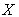be a vector of the nine indicators (observed variables), and then the CFA model can be expressed by: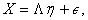(3)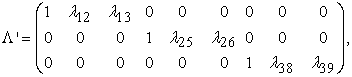where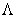is a factor loadings matrix of the vector of latent variables, and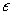is the vector of error. It is assumed that the measurement errors are uncorrelated. The degrees of freedom of model (3) are equal to 24, with 3 parameters fixed to 1 and the other 21 remaining parameters are free to be estimated. Fixing the factor loadings of the first observed variables for each latent variable is done to correctly identify the model. Otherwise, standardizing the variances of the latent variables is the other alternative to identify the model correctly 28.

• Figure 1. Path diagram of the three-factor model for HS39 data
5.2. Normality Check of the Dataset

We will start first by testing the normality of the dataset using MVN package in R.

In Table 5, g1p and g2p are defined as Mardia’s 24 estimation of multivariate skewness and multivariate kurtosis, respectively. Both the skewness (g1p = 6.875188, p-value < 0.05) and kurtosis (g2p = 103.5909, p-value < 0.05) estimates do not indicate multivariate normality, since significant skewness and kurtosis clearly indicate that data are not normal. Therefore, according to Mardia’s MVN test, this data set does not follow a multivariate normal distribution.

In Table 6, H is defined as the value of Royston’s 30 test statistic at significance level 0.05 and p-value is an approximate significance value for the test. According to Royston’s test (H = 125.9724, p-value < 0.05), the data set does not appear to have a multivariate normal distribution. Here, HZ is the value of Henze-Zirkler’s 14 test statistic at significance level 0.05, and p-value is the significance value of this test statistic, in other words it is the significance of multivariate normality. According to the values derived from HZ test (HZ = 1.054447, p-value < 0.05) one can conclude that this multivariate data set deviates also from multivariate normality, since the p-value indicates in significant HZ test statistic.

• Figure 2. Chi-squared QQ plot for HS39 dataset

Sometimes it may occur that MVN test statistic differ from one another, for example HZ test might state normality while the other two tests might result that the data is non-normal. In such cases, it is useful to examine MVN plots along with hypothesis tests in order to reach a more reliable decision 22. A chi-square Q-Q plot was created to examine MVN of HS39 dataset as well. Additionally, Q-Q plot states that if the observed data fit hypothesized distribution, i.e. the points in the Q-Q plot will approximately be on the line it means that the data is normally distributed. Consequently, it is clearly shown in Figure 2 that the points deviate from the line, and therefore dataset does not satisfy MVN assumption.

5.3. Imposing Missing Data

Researchers often confront the problem of missing data in real empirical studies of SEM as well as problem of non-normality. Thus, since these two problems are common in some of SEM studies, it was as important as we shed the light on non-normality in real data to also shed it on missingness problem.

HS39 was used after imposing different rates of missingness using simsem simulation package available in R by Pornprasertmanit et al 27, where a function called imposemissing is used to impose rates of missingness on a simulated data or an existing data frame as in our case, different missingness rates can be imposed either under MCAR or MAR mechanisms.

The same model specification as in section 2 is used here, where a CFA model with three inter-correlated latent variables with three observed indicators each. The estimation procedure is repeated three times with each estimation method under three levels of missingness 5%, 15%, and 30% representing low, moderate and high levels of missingness as seen in Appendix. We chose to implement MCAR technique, since MAR might be in some of the cases neglected, as mentioned by Allison 3 that missing data might be ignored if the data are MAR, since the parameters governing the missing data mechanism are completely distinct from the parameters of the model to be estimated. This somewhat technical condition is unlikely to be violated in the real world.

The three estimation methods known in literature to treat missingness in data were used to fit the model under different rates of missingness. These methods are: FIML, MLR, and Listwise deletion technique using maximum likelihood estimation (ML-LW). Lavaan package was eligible to estimate the model with these three different methods, however, since we were looking mainly to compare between different SEM software AMOS 23 and LISREL 8.8 were used to estimate the model as well but here with only FIML and ML-LW methods as MLR method is not available in them.

5.4. The Results

After conducting the analysis with Lavaan, AMOS, and LISREL, it was found out that the three packages gave out the same parameter estimates with no differences. However, there were only slight differences between the goodness-of-fit indexes for the packages provided as displayed in Table 7.

According to Table 7 we can easily recognize that Lavaan, AMOS, and LISREL packages gave out close if not the similar results of the different indexes with FIML and ML-LW methods. AMOS and LISREL do not report SRMR index under missing cases with FIML method. However, according to the other three indexes it can be easily predicted that it might as well give similar results as in Lavaan. Consequently, we can start comparing directly between the different estimation methods and their performance under different levels of missingness through observing the change that happens in each fit index.

RMSEA

As shown in Table 7, RMSEA values for FIML, MLR, and ML-LW methods were 0.084, 0.055, and 0.055, respectively under 5% missing rate. Contradicting our expectation the increase in missing rates was not reflected negatively on RMSEA values with FIML method as it ranged from 0.084, 0.076, to 0.065 at missingness rates of 5%, 15% till 30%, respectively, reflecting moderate to good model fit. However, both MLR and ML-LW method were affected negatively, for example, RMSEA value of MLR changed from 0.113 to 0.259 at missing rate of 15% to 30%, respectively, and same for ML-LW method reflecting poor to very poor model fit.

SRMR

Lavaan is the only package the reflected SRMR values indicating the following; SRMR values were affected negatively with the increase in the missing rates, and this is across the three estimation methods. For example with FIML method SRMR values were 0.057, 0.063, to 0.065 at missing rates of 5%, 15%, to 30%, respectively, although these changes in the values did not change the status of the model since all of them reflected a good fit to the model. Moreover, MLR method was strongly influenced by the increase in missingness rates compared to FIML in terms of SRMR, as the values ranged from 0.049, 0.080, to 0.188 at missing rates of 5%, 15, and 30%, respectively with model fit changed from good fit to poor or lack of fit at 30% missingness rate. Finally, although ML-LW method was giving good fit at 5% and 15% missing rates, it was strongly influenced with the increase in the missing rates in comparison with the other two estimation methods with SRMR of 0.188 at missing rate 30%.

CFI

CFI measurement was also affected negatively with the increase in missingness rates; however, FIML was slightly affected with this increase in terms of CFI compared to MLR and specifically ML-LW method that was strongly affected especially when the missing rate changed from 15% to 30 %, as its CFI values were 0.976, 0.91, and 0.7 at missing rates of 5%, 15%, and 30%, respectively, where it moved from good fit to a bad fit. Similarly, MLR method followed the same path of ML-LW. Finally, CFI values of FIML method under the three missingness rates indicated a goof fit to the model with slight changes as follows 0.936, 0.936, and 0.938 at missing rates of 5%, 15%, and 30%, respectively, in the three packages.

TLI

Similarly, TLI values reflected the negative impact of the increase in missingness rates on the performance of the estimation methods, except with FIML under lavaan and LISREL it did not show any change in its values. MLR and ML-LW methods were affected by this increase in the missingness rates as they both gave good fit to the model at 5% missing rate with TLI values of MLR and ML-LW equal to 0.966 and 0.964, respectively, and at 15% their TLI values were 0.832 and 0.867, respectively, finally, when missing rate increased to 30% their TLI values declined to 0.556 and 0.550, respectively, indicating poor or lack of model fit.

Generally, Table 7 shows that the three packages indicated similar results for both FIML and ML-LW. Additionally, ML-LW and MLR methods performed equivalently under different rates of missingness, as they were mostly affected by the increase in the missing data rates. Finally, FIML was the best method to deal with different missingness rates.

### 6. Conclusion

In this paper, we reviewed five SEM software packages (AMOS, LISREL, and three packages in R) for dealing with SEM analysis to provide the researcher with a basic guideline about each package. Moreover, we presented an empirical study for assessing the performance of different estimation methods under the existence of missing data.

According to the results, it was found that the conventional techniques such as Listwise generally do a poor job of using only the available information even under any estimation method. However, structural equation modelers are fortunate that many software programs for estimating SEMs now have full information maximum likelihood methods for handling missing data in an optimal fashion 3. This was proved through this study that showed that FIML method outperformed compared to MLR and Listwise deletion (ML-LW) methods; this is because the process works by estimating a likelihood function for each individual based on the variables that are present so that all the available data are used 25. On the other hand, Listwise deletion method seemed to be the mostly affected method by the increase in missingness rates and this was clear through the different used goodness-of-fit indexes, since in this method, the whole case is deleted from the sample if it has missing data on any of the variables in the analysis to be conducted 3, and thus, important information might be disregarded. Thereby, ML-LW method requires sufficiently large sample size especially if the missing rate is above 15%, otherwise it is not recommended. Despite the fact that MLR method is assumed to deal with missing data cases such as MCAR and MAR since it adjusts the goodness-of-fit indexes it uses such as RMSEA, CFI, and TLI to permits MCAR and MAR missing data, again FIML was preferred over MLR method to deal with MCAR missing data.

Finally, it was concluded that the three packages Lavaan, AMOS, and LISREL almost gave out the identical results if the same estimation method is used. Thus, the choice of the used package is left to the researcher’s need and easiness to deal with.

### References

  Abonazel, Mohamed. R. (2014). Statistical analysis using R, Annual Conference on Statistics, Computer Sciences and Operations Research, Vol. 49. Institute of Statistical Studies and research, Cairo University. In article  Abonazel, Mohamed. R. (2015). How to create a Monte Carlo simulation study using R: with Applications on econometric models. Working paper, No. 68708. University Library of Munich, Germany. Online at https://mpra.ub.uni-muenchen.de/68708 In article View Article  Allison, P. D. (2003). Missing data techniques for structural equation modeling. Journal of abnormal psychology, 112(4), 545. In article View Article  PubMed  Arbuckle, J. L. (2010). IBM SPSS Amos 19 user’s guide. Crawfordville, FL: Amos Development Corporation, 635. In article View Article  Bentler, P. M., and Bonett, D. G. (1980). Significance tests and goodness of fit in the analysis of covariance structures. Psychological bulletin, 88(3), 588. In article View Article  Byrne, B. M. (2001). Structural equation modeling with AMOS, EQS, and LISREL: Comparative approaches to testing for the factorial validity of a measuring instrument. International journal of testing, 1(1), 55-86. In article View Article  Byrne, B. M. (2013). Structural equation modeling with AMOS: Basic concepts, applications, and programming. Routledge. In article  Cangur, S., Ercan, I. (2015). Comparison of model fit indices used in structural equation modeling under multivariate normality. Journal of Modern Applied Statistical Methods, 14(1), 152-167. In article View Article  Crawley, M. J. (2012). The R Book, 2nd Ed. John Wiley and Sons Ltd. In article View Article  El-Sheikh, Ahmed A., Abonazel, Mohamed R., Gamil, Noha (2017). A review of estimation methods for structural equation modeling. Working paper. Institute of Statistical Studies and Research. Cairo University, Egypt. In article  Enders, C. K., and Bandalos, D. L. (2001). The relative performance of full information maximum likelihood estimation for missing data in structural equation models. Structural Equation Modeling, 8(3), 430-457. In article View Article  Fox, J. (2006). Teacher's corner: structural equation modeling with the sem package in R. Structural equation modeling, 13(3), 465-486. In article View Article  Gamil, Noha (2017). Estimation methods of structural equation Models: A comparative study. MSc thesis. Institute of Statistical Studies and Research. Cairo University. In article  Henze, N., and Zirkler, B. (1990). A class of invariant consistent tests for multivariate normality. Communications in Statistics-Theory and Methods, 19(10), 3595-3617. In article View Article  Hooper, D., Coughlan, J., and Mullen, M. R. (2008). Structural Equation Modelling: Guidelines for Determining Model Fit. Electronic Journal of Business Research Methods, 6(1), 53-60. In article View Article  Hox, J. J., Maas, C. J., and Brinkhuis, M. J. (2010). The effect of estimation method and sample size in multilevel structural equation modeling. StatisticaNeerlandica, 64(2), 157-170. In article View Article  Hoyle, R. H. (2012). Handbook of structural equation modeling. Guilford Press. In article View Article  Hu, L. T., and Bentler, P. M. (1999). Cutoff criteria for fit indexes in covariance structure analysis: Conventional criteria versus new alternatives. Structural equation modeling: a multidisciplinary journal, 6(1), 1-55. In article View Article  Iacobucci, D. (2010). Structural equations modeling: Fit indices, sample size, and advanced topics. Journal of Consumer Psychology, 20(1), 90-98. In article View Article  Jöreskog, K. G., and Sörbom, D. (1993). LISREL 8: Structural equation modeling with the SIMPLIS command language. Scientific Software International. In article View Article  Kline, Rex B. (2011). Principles and practice of structural equation modeling. (3rded). New York: The Guilford Press. In article View Article  Korkmaz, S., Goksuluk, D., and Zararsiz, G. (2014). MVN: an R package for assessing multivariate normality. The R Journal, 6(2), 151-162. In article View Article  MacCallum, R. C., Browne, M. W., and Sugawara, H. M. (1996). Power analysis and determination of sample size for covariance structure modeling. Psychological methods, 1(2), 130. In article View Article  Mardia, K. V. (1970). Measures of multivariate skewness and kurtosis with applications. Biometrika, 519-530. In article View Article  Newsom, J. T. (2015). Some clarifications and recommendations on fit indices. USP, 655. In article  Olsson, U. H., Foss, T., Troye, S. V., and Howell, R. D. (2000). The performance of ML, GLS, and WLS estimation in structural equation modeling under conditions of misspecification and nonnormality. Structural equation modeling, 7(4), 557-595. In article View Article  Pornprasertmanit, S., Miller, P., Schoemann, A., Quick, C., Jorgensen, T., and Pornprasertmanit, M. S. (2016). Package ‘simsem’. In article  Rosseel, Y. (2012). lavaan: An R package for structural equation modeling. Journal of Statistical Software, 48(2), 1-36. In article View Article  Rosseel, Y., Oberski, D., Byrnes, J., Vanbrabant, L., Savalei, V., Merkle, E., and Rosseel, M. Y. (2015). Package ‘lavaan’. In article  Royston, J. P. (1983). Some techniques for assessing multivarate normality based on the Shapiro-Wilk W. Applied Statistics, 121-133. In article View Article

### Appendix: R-codes

library("lavaan"); library("simsem"); library("MVN")

##--- The data

hsdata<-HolzingerSwineford1939

##--- Normality Check

###------------------------

x<-hsdata[,7:15]

mardiaTest(x,qqplot=TRUE)

roystonTest(x,qqplot=TRUE)

hzTest(x,qqplot=TRUE)

##--- Building the model

##----------------------------

HSmodel<- '

visual=~x1+x2+x3

textual=~x4+x5+x6

speed=~x7+x8+x9

'

##--- impose missing rates

##-------------------------------

miss.data1<-imposeMissing(hsdata,pmMCAR =0.05)

miss.data2<-imposeMissing(hsdata,pmMCAR =0.15)

miss.data3<-imposeMissing(hsdata,pmMCAR =0.30)

##--- fitting the model using ML-LW, MLR, and FIML methods

##-------------------------------------------------------------------

##--- imposing 5% missing values

##---------------------------------------

Fit.L<-cfa(HSmodel, data=miss.data1,missing="listwise")

summary(Fit.L, fit.measures=TRUE, standardized=TRUE)

Fit.M<-cfa(HSmodel, data=miss.data1,estimator="MLR")

summary(Fit.M, fit.measures=TRUE, standardized=TRUE)

Fit.fiml<-cfa(HSmodel, data=miss.data1,missing="fiml")

summary(Fit.fiml, fit.measures=TRUE, standardized=TRUE)

##--- imposing 15% missing values

##----------------------------------------

Fit.L<-cfa(HSmodel, data=miss.data2,missing="listwise")

summary(Fit.L, fit.measures=TRUE, standardized=TRUE)

Fit.M<-cfa(HSmodel, data=miss.data2,estimator="MLR")

summary(Fit.M, fit.measures=TRUE, standardized=TRUE)

Fit.fiml<-cfa(HSmodel, data=miss.data2,missing="fiml")

summary(Fit.fiml, fit.measures=TRUE, standardized=TRUE)

##--- imposing 30% missing values

##------------------------------------------

Fit.L<-cfa(HSmodel, data=miss.data3,missing="listwise")

summary(Fit.L, fit.measures=TRUE, standardized=TRUE)

Fit.M<-cfa(HSmodel, data=miss.data3,estimator="MLR")

summary(Fit.M, fit.measures=TRUE, standardized=TRUE)

Fit.fiml<-cfa(HSmodel, data=miss.data3,missing="fiml")

summary(Fit.fiml, fit.measures=TRUE, standardized=TRUE)This work is licensed under a Creative Commons Attribution 4.0 International License. To view a copy of this license, visit http://creativecommons.org/licenses/by/4.0/